Free Printable Worksheets Covering Addition and Subtraction with Whole Numbers and Fractions

## Fraction Subtraction with Same Denominators (Set 2)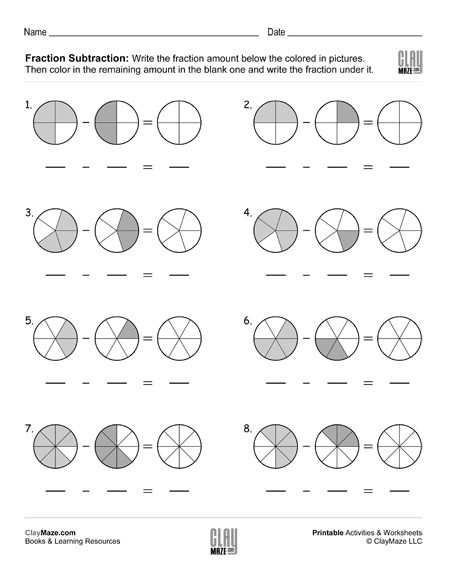Fraction subtraction with common denominators – write the fractions, then subtract them using the pictures and color in the correct amount....

## Fraction Subtraction with Same Denominators (Set 3)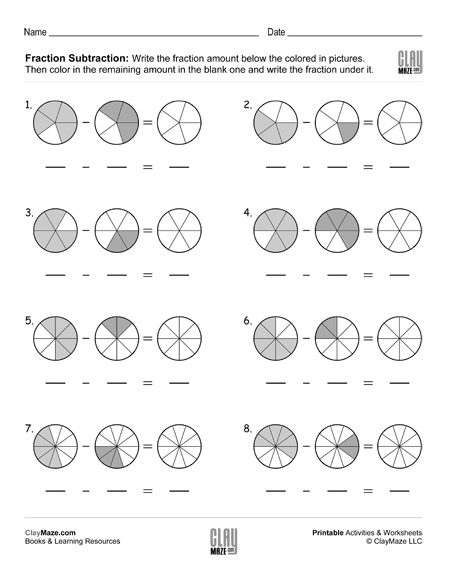Subtracting fractions free, printable worksheet – Write the fractions, subtract them and color the remaining amount....

## Fraction Subtraction with Same Denominators (Set 4)Fraction subtraction with common denominators worksheet – Write the correct fraction amount under each picture. Subtract the fractions and color the fractional amount in the blank picture....

## Fraction Addition and Simplifying with Coloring (Set 1)Add the fractions and color the correct amount. Simplify the sum....

## Fraction Addition and Simplifying with Coloring (Set 2)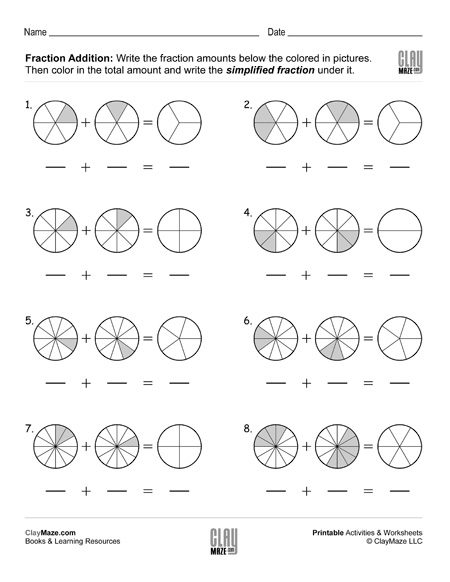Add the fractions and color the correct amount. Simplify the sum....

## Subtraction Facts Practice Worksheet (Set 1)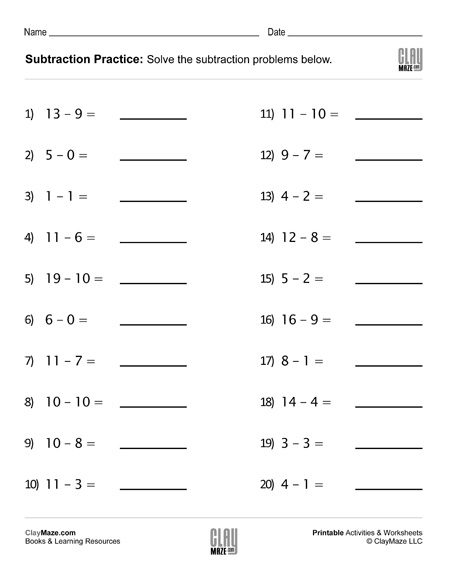Help your child learn and remember the subtraction facts with our subtraction facts worksheets – 20 problems....

## Subtraction Facts Practice Worksheet (Set 2)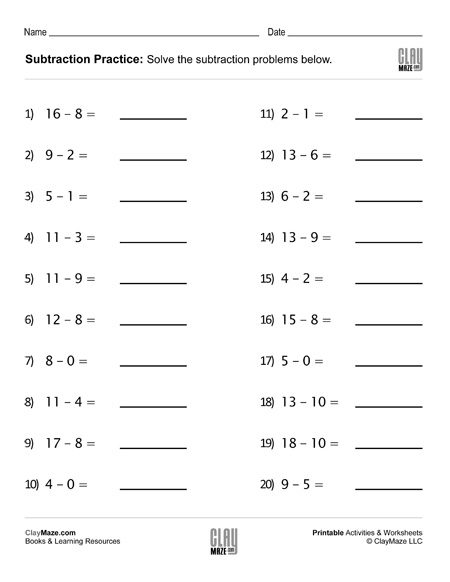Practice the subtraction facts with our subtraction facts worksheets – 20 problems....

## Subtraction Facts Practice Worksheet (Set 3)Practice the subtraction facts with our subtraction drills – 20 problems....

## Subtraction Facts Practice Worksheet (Set 4)Free worksheet to help learn the subtraction facts – 20 problems....

## Addition Facts Practice Worksheet (Set 1)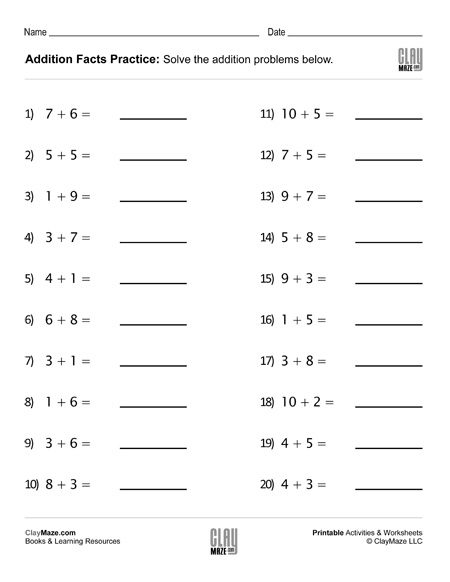## Addition Facts Practice Worksheet (Set 2)Practice for addition facts. – 20 problems...

## Addition Facts Practice Worksheet (Set 3)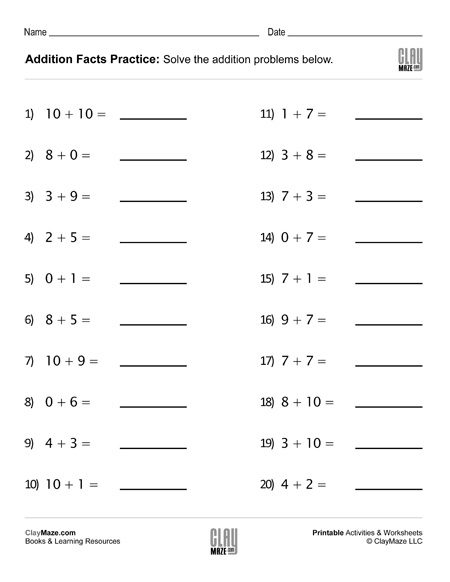Free addition facts worksheet. – 20 problems...

## Addition Facts Practice Worksheet (Set 4)Practice the addition facts with this worksheet – 20 problems....

## Find the Missing Addends Worksheet (Set 1)Free Math Worksheet – Find the Missing Addends – 20 problems...

## Find the Missing Addends Worksheet (Set 2)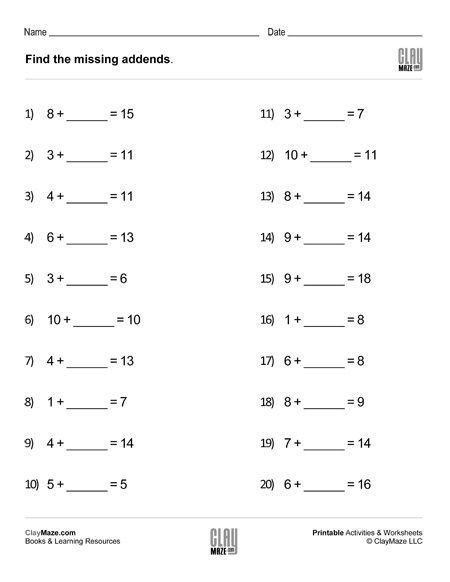Worksheet for addition and subtraction practice. – 20 problems...